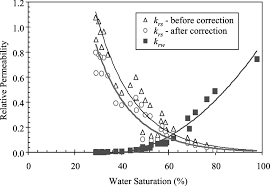## How to Calculate and Solve for Permeability | Mass TransferThe image above represents permeability.

To compute for permeability, five essential parameters are needed and these parameters are Constant (A), Partial Pressure (Po), Activation due to Permeation (Qp), Gas Constant (R) and Temperature (T).

The formula for calculating permeability:

P = APo0.5 . e-Qp/RT

Where:

P = Permeability
A = Constant
Po = Partial Pressure
Qp = Activation due to Permeation
R = Gas Constant
T = Temperature

Let’s solve an example;
Find the permeability when the constant is 12, the partial pressure is 22, the activation due to permeation is 10, the gas constant is 14 and the temperature is 2.

This implies that;

A = Constant = 12
Po = Partial Pressure = 22
Qp = Activation due to Permeation = 10
R = Gas Constant = 14
T = Temperature = 2

P = APo0.5 . e-Qp/RT
P = 12(22)0.5 . e-(10)/(14)(2)
P = 12(4.69) . e-10/28
P = 56.28 . e-0.357
P = 56.28 (0.699)
P = 39.38

Therefore, the permeability is 39.38.